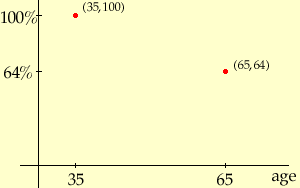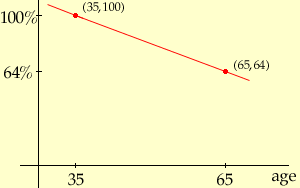Name: gayle Who is asking: Parent Level: Middle Question: I don't understand the second part of this problem Here is the first part that was correct. A 35yr old has 100% blood flow. A 65yr old has 64% blood flow. Find the rate of change per yr in the percent of blood flow. (This part is correct) m=(64-100)/(35-65)= -36/30= -1.2 per year of blood flow. Now, here is the second part I DON'T UNDERSTAND! .Let b represent the percent of blood flow and a represent a person's age. Write an equation that you could use to predict the percent of blood flow for a person of any age over 35. Hi Gayle, I would plot a graph showing age on the horizontal axis and percent of blood flow on the vertical axis. Put the two points that you know on the graph. (When a = 35 Years, b = 100% and when a = 65 Years, b = 64%.)The equation that you want is the equation that describes the line joining these two points. This line has equation b = ma + k where m is the slope that you found in the first part of the problem, that is m = -1.2 so b = -1.2a + k.You can find k by substituting one of the points into the equation. Substituting a = 35 and b = 100 gives 100 = -1.2(35) + k or 100 = -42 + k Thus k = 142 and the equation is b = -1.2a + 142 I hope this helps, Penny Go to Math Central